On-line GuidesAll GuideseBook StoreiOS / AndroidLinux for BeginnersOffice ProductivityLinux InstallationLinux SecurityLinux UtilitiesLinux VirtualizationLinux KernelSystem/Network AdminProgrammingScripting LanguagesDevelopment ToolsWeb DevelopmentGUI Toolkits/DesktopDatabasesMail SystemsopenSolarisEclipse DocumentationTechotopia.comVirtuatopia.comHow To GuidesVirtualizationGeneral System AdminLinux SecurityLinux FilesystemsWeb ServersGraphics & DesktopPC HardwareWindowsProblem SolutionsPrivacy Policy## Digression on The Sigma Operator

For those programmers new to statistics, this section provides background on the Sigma operator, Σ.

Equation 13.3. Basic Summation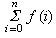The Σ operator has three parts to it. Below it is a bound variable, i and the starting value for the range, written as i =0. Above it is the ending value for the range, usually something like n . To the right is some function to execute for each value of the bound variable. In this case, a generic function, f ( i ). This is read as “sum f ( i ) for i in the range 0 to n ”.

One common definition of Σ uses a closed range, including the end values of 0 and n . However, since this is not a helpful definition for software, we will define Σ to use a half-open interval. It has exactly n elements, including 0 and n -1; mathematically,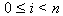.

Consequently, we prefer the following notation, but it is not often used. Most statistical and mathematical texts seem to use 1-based indexing, consequently some care is required when translating formulae to programming languages that use 0-based indexing.

Equation 13.4. Summation with Half-Open Interval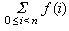Our two statistical algorithms have a form more like the following. In this we are applying some function, f (), to each value, xi of an array. When computing the mean, there is no function. When computing standard deviation, the function involves subtracting and multiplying.

Equation 13.5. Summing Elements of an Array, x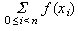We can transform this definition directly into a for loop that sets the bound variable to all of the values in the range, and does some processing on each value of the `list` of integers. This is the Python implemention of Sigma. This computes two values, the sum, `sum`, and the number of elements, `n`.

Example 13.2. Python Sigma Iteration

```sum= 0
for i in range(len(theList)):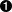xi= theList[i]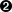# fxi = processing of xi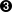sum += fxi
n= len(theList)
```Get the length of `theList`. Execute the body of the loop for all values of `i` in the range 0 to the number of elements-1.Fetch item `i` from `theList` and assign it to `xi`.For simple mean calculation, this statement does nothing. For standard deviation, however, this statement computes the measure of deviation from the average.

More advanced Python programmers may be aware that there are several ways to shorten this loop down to a single expression using the `reduce` function as well as list comprehensions.

An Optimization. In the usual mathematical notation, an integer index, i is used. In Python it isn't necessary to use the formal integer index. Instead, an iterator can be used to visit each element of the `list`, without actually using an explicit numeric counter. The processing simplifies to the following.

Example 13.3. Python Sample Values by Iterator

```for xi in theList:# fxi = processing of xisum += fxi
n= len(theList)
```Execute the loop assigning each item of of `theList` to `xi`.For simple mean calculation, this statement does nothing. For standard deviation, however, this statement computes the measure of deviation from the average.Published under the terms of the Open Publication License Design by Interspire